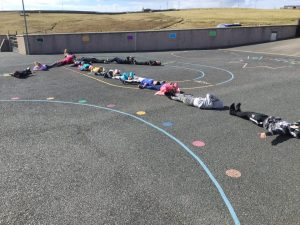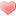Outdoor learning – maths

Today we took advantage of the beautiful weather and went outside to do a little revision for our maths lesson. The pupils had to work together to use their bodies to answer the maths question. They had to show an understanding of the maths task and work well within their team to get themselves in the correct position to provide the correct answer – sometimes easier said than done…

Firstly we described a 2D shape.
A rectangle –

An equilateral triangle –

A pentagon with only person speaking to position bodies –

A maths sum where the answer was 4 –

A maths sum where the answer is 10 –

A maths sum where the answer is 14 –

The answer is 15 and 102 –

Just for fun we joined together to make a big wind turbine.We had a lot of fun in our maths lesson today!(5 votes, average: 1.00 out of 1)Loading...

One thought on “Outdoor learning – maths”

1.Yvonne Tait says:

It looks like a lot of fun! 🙂